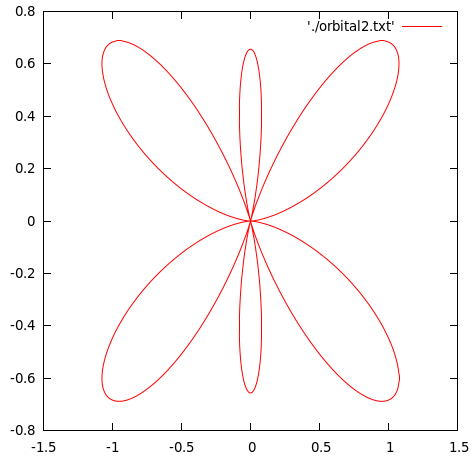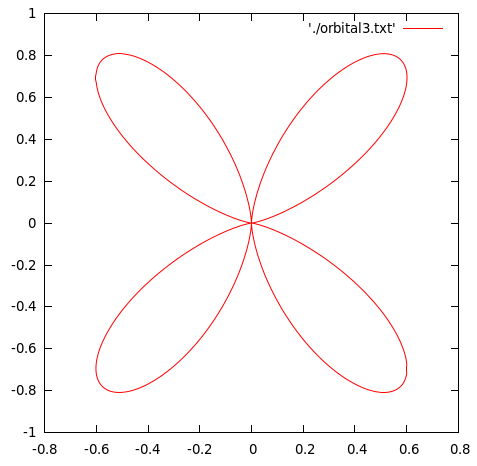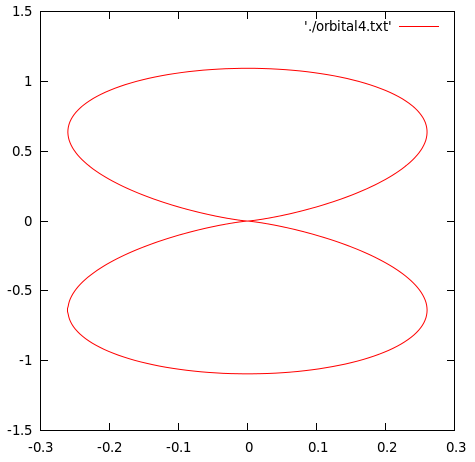# Plotting exercises for C and GNUPlot

Gnuplot is a portable command-line driven graphing utility for Linux and other OS.
C and Gnuplot can be used to plot complex functions.

One can write the function in C and then write the values of the function at various values in a txt file, which can then be plotted using Gnuplot.
The txt file should have numerical values in at least two columns. The first column is for x values. The rest of the columns are for y-axis values.

Following are some of the exercises to help you understand the process in a better way.

### Plot a circle of a given radius and center using C and Gnuplot.

A. We can do this by writing a C program that calculates the x and y-values of the required circle and then write those values in a txt file. Then we can plot the file using Gnuplot.

#### Program:

/*************************************
***********PLOT A CIRCLE ************
************************************/
#include<stdio.h>
#include<math.h>
main(){
FILE *fp=NULL;
fp=fopen("circle.txt","w");
double r;
double x,y,x0,y0;
printf("Enter the radius of the circle to be plotted: ");
scanf("%lf",&r);
printf("Enter the x and y-coordinates of the center: ");
scanf("%lf%lf",&x0,&y0);
for(y=y0-r;y<=y0+r;y=y+0.1){
x=sqrt(r*r-(y-y0)*(y-y0))+x0;
fprintf(fp,"%lf\t %lf\n",x,y);
}
for(y=y0+r;y>=y0-r;y=y-0.1){
x=-sqrt(r*r-(y-y0)*(y-y0))+x0;
fprintf(fp,"%lf\t %lf\n",x,y);

}
}


The above program will generate a txt file(circle.txt) with the x and y-values for the circle of required radius and center coordinates.
Then the plotting can be done using Gnuplot by using the following command:
plot 'circle.txt' w l

### OUTPUT:### Plot$|\Theta_{lm}(\theta)|^2$, the square modulus of the orbital wave function for$l = 3;m = 0,\pm1,\pm2,\pm3$. The values of$|\Theta_{lm}(\theta)|^2$ are given by$\Theta_{3,0}(\theta)=\frac{3\sqrt{14}}{4}\left(\frac{5}{3}\cos^3\theta-\cos\theta\right)$$\Theta_{3,\pm1}(\theta)=\frac{\sqrt{42}}{8}\sin\theta\left(5\cos^2\theta-1\right)$$\Theta_{3,\pm2}(\theta)=\frac{\sqrt{105}}{4}\sin^2\theta\cos\theta$$\Theta_{3,\pm3}(\theta)=\frac{\sqrt{70}}{8}\sin^3\theta$

Solution:

#### PROGRAM:

/**************************************
******PLOT ORBITAL WAVEFUNCTIONS******
*************************************/
#include<stdio.h>
#include<math.h>
double theta30(double x){
double out=3.0*sqrt(14.0)/4.0*(5.0/3.0*pow(cos(x),3)-cos(x));
return out;
}
double theta31(double x){
double out=(sqrt(42))/(8)*sin(x)*(5*pow(cos(x),2)-1);
return out;
}
double theta32(double x){
double out=sqrt(105)/4*pow(sin(x),2)*cos(x);
return out;
}
double theta33(double x){
double out=(sqrt(70))/(8)*(pow(sin(x),3));
return out;
}
main(){
double theta;
double x1,x2,x3,x4,y1,y2,y3,y4;
FILE *fp1=NULL;
FILE *fp2=NULL;
FILE *fp3=NULL;
FILE *fp4=NULL;
fp1=fopen("orbital1.txt","w");
fp2=fopen("orbital2.txt","w");
fp3=fopen("orbital3.txt","w");
fp4=fopen("orbital4.txt","w");
for(theta=0;theta<=2*M_PI;theta=theta+0.01){
x1=theta30(theta)*theta30(theta)*cos(theta);
x2=theta31(theta)*theta31(theta)*cos(theta);
x3=theta32(theta)*theta32(theta)*cos(theta);
x4=theta33(theta)*theta33(theta)*cos(theta);
y1=theta30(theta)*theta30(theta)*sin(theta);
y2=theta31(theta)*theta31(theta)*sin(theta);
y3=theta32(theta)*theta32(theta)*sin(theta);
y4=theta33(theta)*theta33(theta)*sin(theta);
fprintf(fp1,"%lf\t%lf\n",x1,y1);
fprintf(fp2,"%lf\t%lf\n",x2,y2);
fprintf(fp3,"%lf\t%lf\n",x3,y3);
fprintf(fp4,"%lf\t%lf\n",x4,y4);
}
}


The above program would generate for txt files containing the data-points for the four orbital equations(orbital1.txt,orbital2.tx,….). These can then be plotted using Gnuplot by using the following command:
plot 'orbital1.txt' w l

### OUTPUT:$\Theta_{3,0}(\theta)=\frac{3\sqrt{14}}{4}\left(\frac{5}{3}\cos^3\theta-\cos\theta\right)$$\Theta_{3,\pm1}(\theta)=\frac{\sqrt{42}}{8}\sin\theta\left(5\cos^2\theta-1\right)$$\Theta_{3,\pm2}(\theta)=\frac{\sqrt{105}}{4}\sin^2\theta\cos\theta$$\Theta_{3,\pm3}(\theta)=\frac{\sqrt{70}}{8}\sin^3\theta$### REFERENCES:

The above problems have been taken from the Computer Programming & Numerical Analysis Manual by Dr. Shobhit Mahajan.## One thought on “Plotting exercises for C and GNUPlot”

1. Do you have anything related to finding the Jump Discontinuities of a plot in C programming.